# 动态稳定性参数风洞实验支撑干扰数值模拟研究Numerical Simulation of Support Interference in Wind Tunnel for Dynamic Stability Parameters

DOI: 10.12677/IJFD.2019.71005, PDF, HTML, XML, 下载: 504  浏览: 2,079

Abstract: In hypersonic wind tunnel, sting support is usually used for the special test of dynamic stability parameters. A Computational Fluid Dynamics analysis of the dynamics of airbreathing hypersonic airframe/propulsion integrative vehicle at supersonic flight conditions is performed using 3D un-steady Navier Stokes equations. The 2-order Roe scheme and the dual-time-step method based on LU-SGS are respectively applied to discrete of the spatial and time derivative of the unsteady flow. Based on the unsteady Etkin pneumatics model, this analysis uses dynamic force-harmonic simu-lations to develop direct-dynamic stability derivatives, as well as cross and cross-coupling deriva-tives. Numerical simulation of 7˚ blunt cone model was carried out by three different sting supports. On the basis of standard model verification, the influence of sting interference on dynamic derivatives was analyzed, so as to get the dynamic derivative variations brought by the sting in-terference.

1. 引言

2. 数值方法

$\frac{\partial U}{\partial t}+\frac{\partial E}{\partial \xi }+\frac{\partial F}{\partial \eta }+\frac{\partial G}{\partial \zeta }=\frac{\partial {E}_{v}}{\partial \xi }+\frac{\partial {F}_{v}}{\partial \eta }+\frac{\partial {G}_{v}}{\partial \zeta }$ (1)

3. 动导数计算方法Table 1. Dynamic damping derivatives

${C}_{m}={C}_{m}\left(\alpha \left(t\right),\stackrel{˙}{\alpha }\left(t\right),\stackrel{¨}{\alpha }\left(t\right),\cdots ,q\left(t\right),\stackrel{˙}{q}\left(t\right),\stackrel{¨}{q}\left(t\right),\cdots \right)$ (2)

${C}_{mq}+{C}_{m\stackrel{˙}{\alpha }}=\frac{1}{{\alpha }_{m}\pi }{\int }_{{t}_{s}}^{{t}_{s}+{T}_{c}}{C}_{m}\left(t\right)\mathrm{cos}ktdt$ (3)

4. 验证算例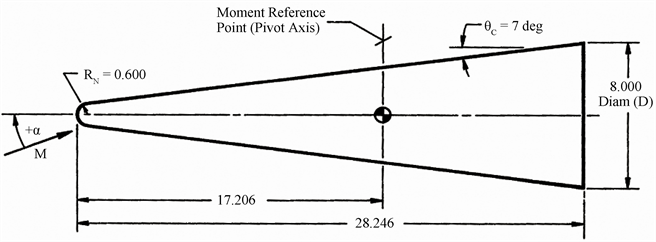Figure 1. Blunt cone dimensions and centroid position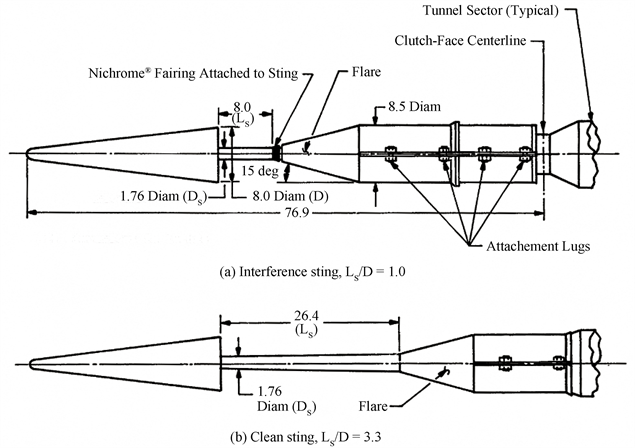Figure 2. Two forms of support for the blunt cone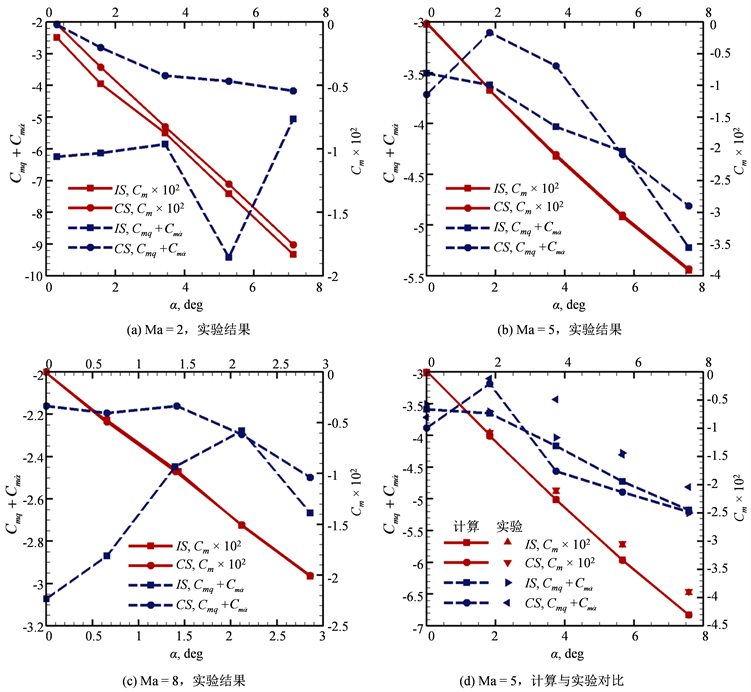Figure 3. Comparison of moment coefficients of two support forms

5. 类X-51A支撑干扰计算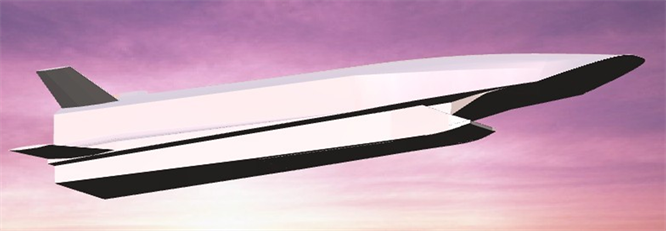Figure 4. X-51-like hypersonic vehicleFigure 5. Internal flow channel outline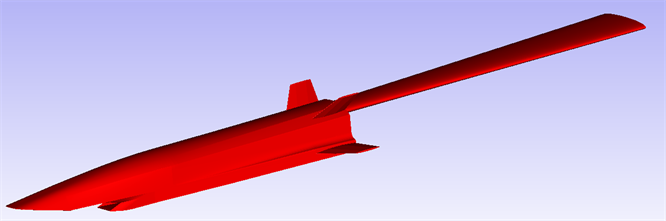Figure 6. Sting support outlineTable 2. Comparison between the real shape and the experi-mental shape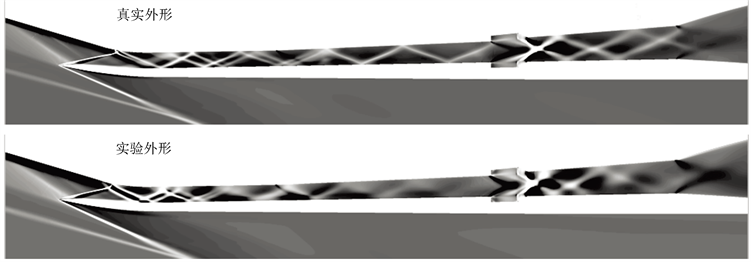Figure 7. Schlieren photograph of inlet symmetry planeFigure 8. Pitch moment coefficient and inlet performance parameters loop

6. 结论

  Loeser, T. and Rohlf, D. (2016) Experimental Determination of Dynamic Derivatives in a Wind Tunnel Using Parameter Identification. Springer International Publishing, Berlin.  Uselton, J.C. and Uselton, B.L. (2015) Validity of Small-Amplitude Oscillation Dynamic-Stability Measurement Technique. Journal of Spacecraft & Rockets, 13, 266-270. https://doi.org/10.2514/3.57086  Liu, X., Liu, W., Chai, Z. and Yang, X. (2016) Research Progress of Numerical Method of Dynamic Stability Derivatives of Aircraft. Acta Aeronautica et Astronautica Sinica, 37, 2348-2369.  Mackay, M. (1993) A Review of Sting Support Interference and Some Related Issues for the Marine Dynamic Test Facility (MDTF). Defense Research Reports.  Ericsson, L. and Reding, J. (1983) Review of Support Interference in Dynamic Tests. AIAA Journal, 21, 1652-1666. https://doi.org/10.2514/3.60166  Taylor, G. and Gursul, I. (2005) Support Interference for a Maneuvering Delta Wing. Journal of Aircraft, 42, 1504-1515. https://doi.org/10.2514/1.12094  Yi, B., Zeng, K. and Wang, S. (2016) Investigation on the Interference Correction of Flying Wing Aircraft Rear Sting Support in High Speed Wind Tunnel Test. ACTA Aerodynamica Sinica, 34, 98-106.  李周复, 风洞试验手册[M]. 北京: 航空工业出版社, 2015.  Zhang, M., Dai, J. and Chen, N. (2016) Transient Dynamic Stability Test Method for Shock Wind Tunnel. Journal of Electronic Measurement & Instrumentation, 30.  郭林亮, 祝明红, 孔鹏, 等. 风洞虚拟飞行模型机与原型机动力学特性分析[J], 航空学报, 2016, 37(8): 2583-2593.  Pan, J., Zhang, J., Cai, Y. and Xu, M. (2016) Investigation on the High-Speed Wind Tunnel Dynamic Derivative Test Accuracy Promotion. Acta Aerodynamica Sinica, 34, 606-610.  刘伟, 赵海洋, 杨小亮. 飞行器动态气动特性数值模拟方法[M], 长沙: 国防科技大学出版社, 2015.  Mi, B., Zhan, H. and Chen, B. (2017) Calculating Dynamic Derivatives of Flight Vehicle with New Engi-neering Strategies. International Journal of Aeronautical & Space Sciences, 18, 175-185. https://doi.org/10.5139/IJASS.2017.18.2.175  刘绪, 赵云飞, 王东方, 等. 高超声速内外流一体化飞行器动态特性[J]. 弹道学报, 2013, 25(3): 38-43.  刘绪. 高超声速内外流一体化飞行器动态特性研究[D]; [硕士学位论文]. 长沙: 国防科学技术大学, 2011.  Uselton, B.L. and Cyran, F.B. (1980) Sting Interference Effects as Determined by Measurements of Dynamic Stability Derivatives, Surface Pressure, and Base Pressure for Mach Numbers 2 through 8. DTIC Document.